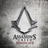8Cangtou 最近的时间轴更新# 8Cangtou

V2EX 第 233965 号会员，加入于 2017-06-05 11:58:19 +08:008Cangtou 创建的所有主题提问技术话题好玩工作信息交易信息城市相关根据 8Cangtou 的设置，主题列表被隐藏
8Cangtou 最近回复了
 26 天前 回复了 carolv 创建的主题 › 2020 › 大家跨年怎么过？

 76 天前 回复了 wanacry 创建的主题 › 奇思妙想 › 盲人可以玩王者荣耀吗

 90 天前 回复了 gdw1986 创建的主题 › Python › 估计面试没通过，唉

 102 天前 回复了 jzyff 创建的主题 › 程序员 › 大家写业务代码有什么心得吗？

 103 天前 回复了 zzzrf 创建的主题 › LeetCode › Google 面试题：搜索二维矩阵 II
func searchMatrix (matrix [][]int, target int) int {
var count int
for i := 0; i < len(matrix); i++{
if target < matrix[i] || target > matrix[i][len(matrix[i])-1]{
continue
}
if binary_search(matrix[i], target){
count++
}
}
return count
}

func binary_search(nums []int, target int) bool{
begin := 0
end := len(nums) - 1
for begin + 1 < end{
mid := begin + (end - begin) / 2
if nums[mid] > target{
end = mid
}else if nums[mid] < target{
begin = mid
}else {
return true
}
}

if nums[begin] == target{
return true
}else if nums[end] == target{
return true
}else {
return false
}
}
 132 天前 回复了 zzzrf 创建的主题 › 推广 › 巨硬面试题：最大子数组
func maxSubArray(nums []int) int {
if len(nums) == 1{
return nums
}
dp := make([]int, len(nums))
dp = nums
res := nums
for i := 1;i < len(nums); i++{
dp[i] = max(dp[i-1] + nums[i], nums[i])
res = max(res, dp[i])
}
return res
}

func max(a, b int) int{
if a > b{
return a
}
return b
}
 150 天前
@Reol 棋牌游戏公司= =
 150 天前

 286 天前 回复了 tyhuohuo8 创建的主题 › 问与答 › 大家的小学，初中，同学还联系的多吗？